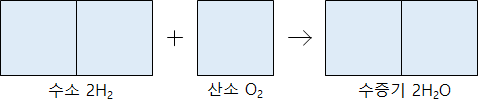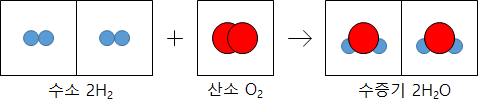# Chemical Reactions in Gas Phase – Water(Steam) Synthesis

Chemical Reactions in Gas Phase

Hydrogen gas and oxygen gas react to produce water vapor. At this time, the hydrogen gas and the oxygen gas react at a volume ratio of 2: 1 to generate 2 volumes of water vapor.When the gas reacts at a constant temperature and pressure and a new gas is produced, there is always a simple integer ratio between the volumes of each gas.

The reason why the volume ratio is expressed as a simple integer ratio is that the number of molecules in the same volume of gas is the same at the same temperature and pressure regardless of the gas type. In 1811, Avogadro, an Italian scientist, published it as a rule.That is, the ratio of the coefficients is the same as the ratio of the number of molecules in the chemical reaction formula in which the gas reacts, and the ratio of the number of molecules is equal to the volume ratio.